CHORD CHART

Online application
by Yann Ics

2015 – 2021

fill in the needed fields in the following form :Mind that the result could take some time ... so be patient.

Or upload a chordChart file (max size 100 ko) :

Mind that the result could take some time ... so be patient.

Tutorial: ./chordChart/tuto.html
Reference: https://yannics.github.io
Contact: by.cmsc@gmail.com

 \a\la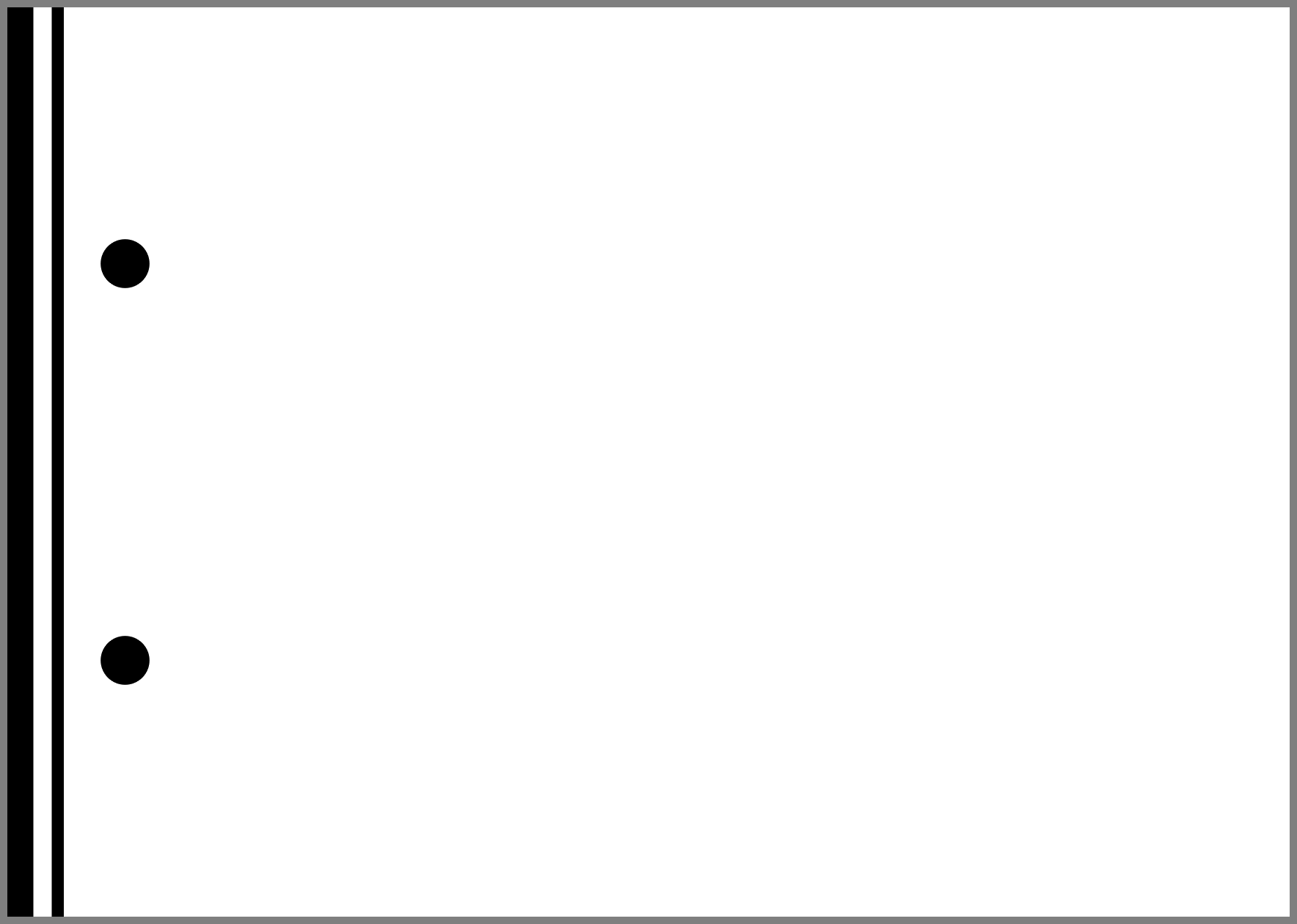w w \b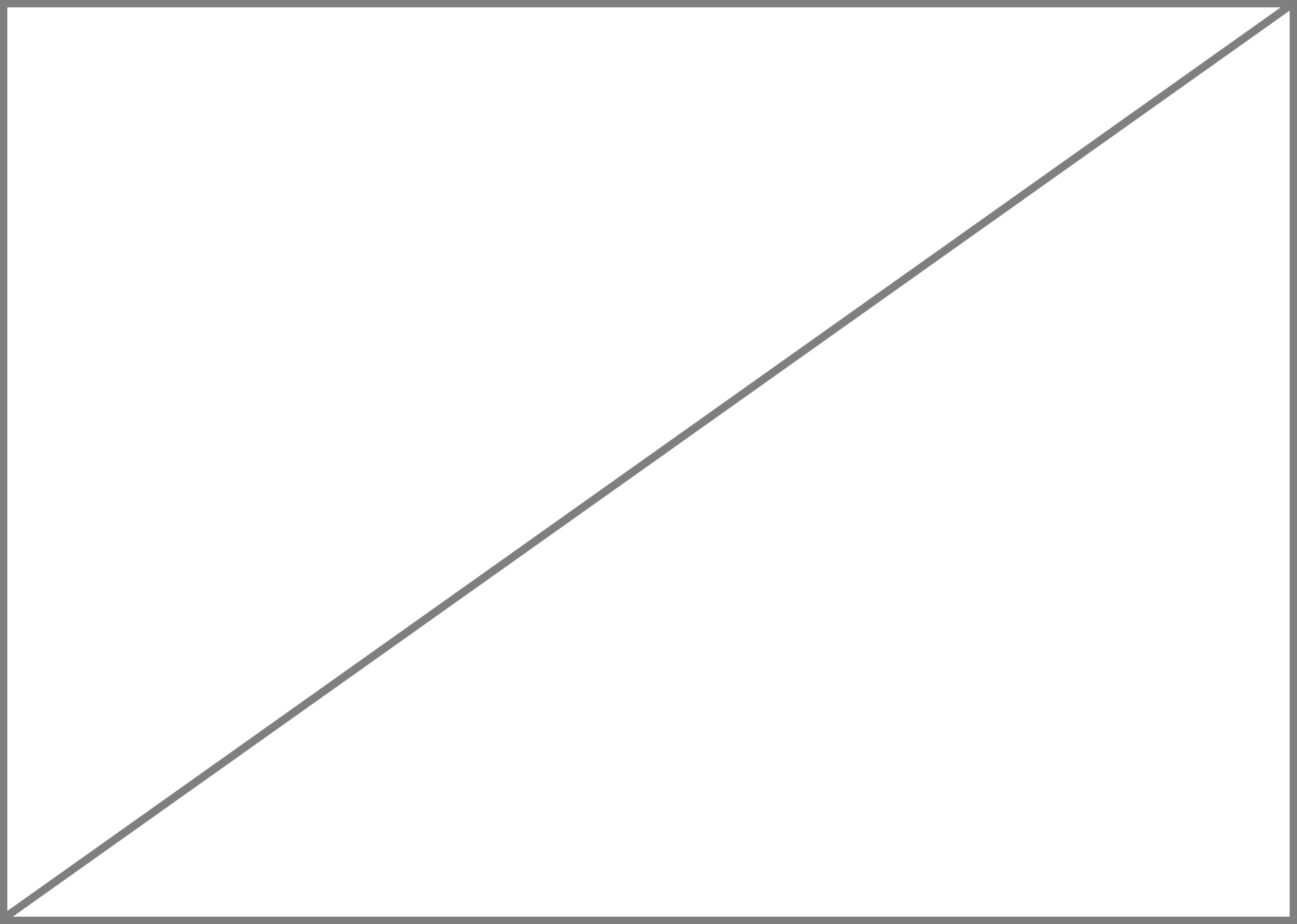\lb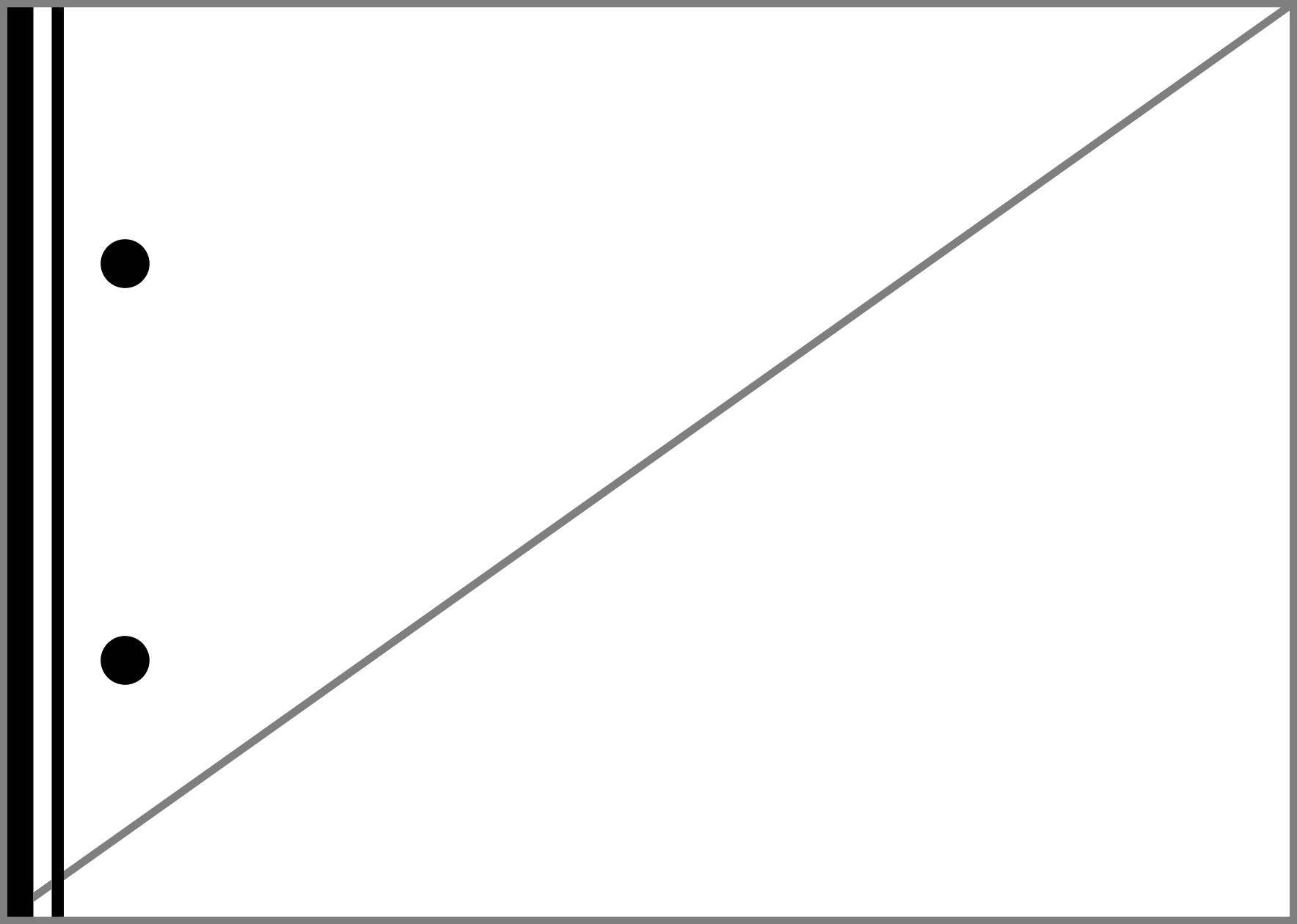W W \c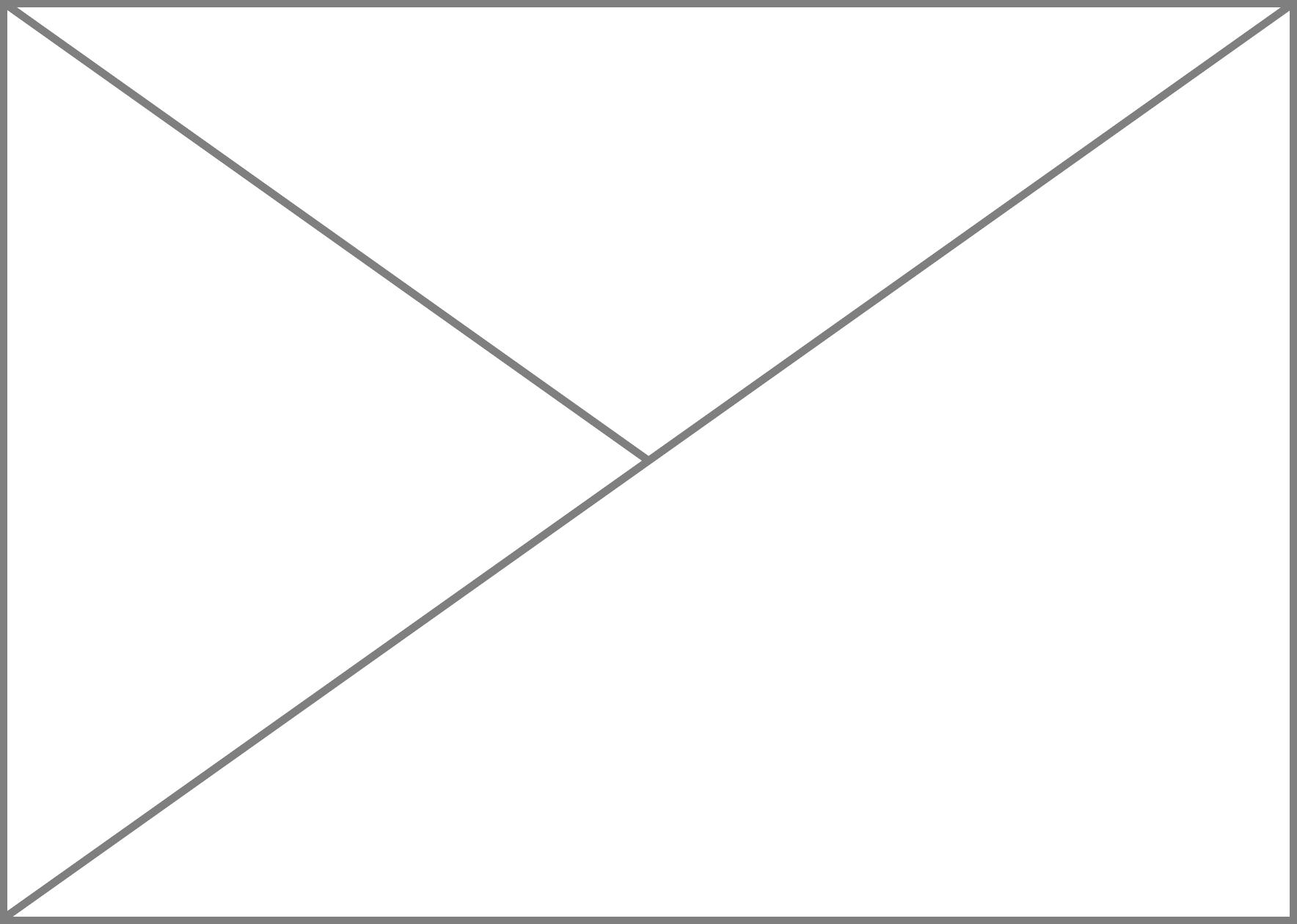\lc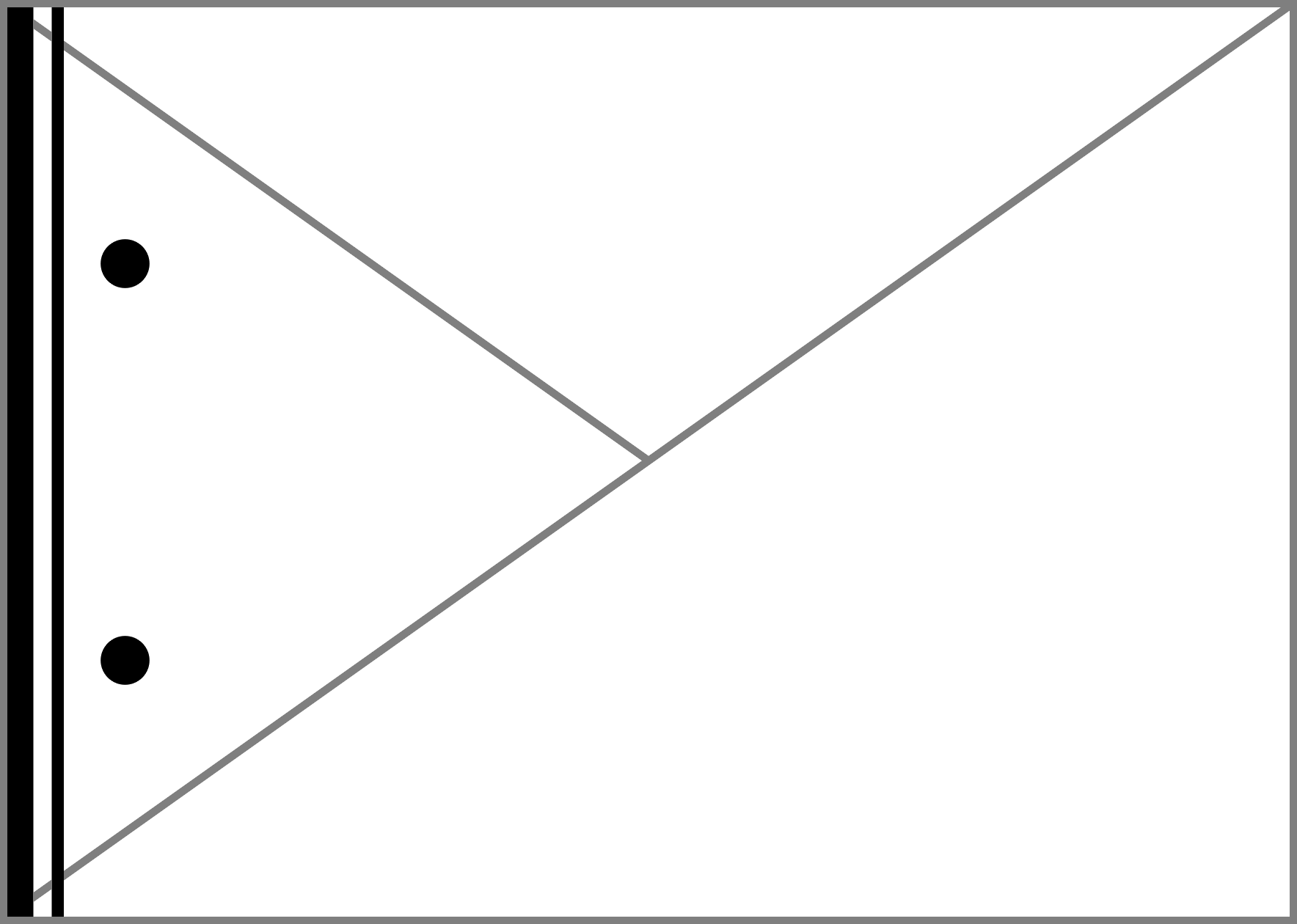# # \d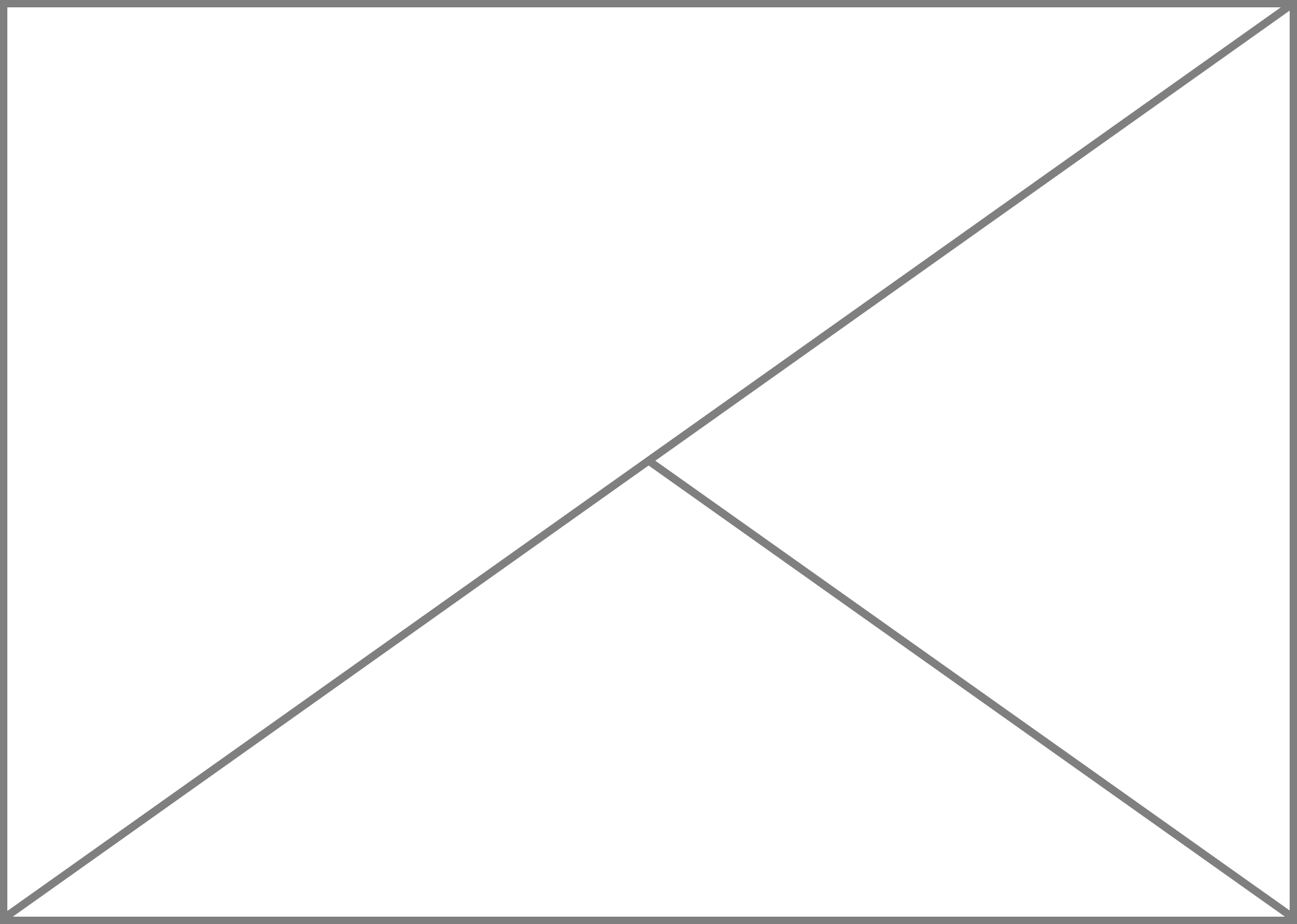\ld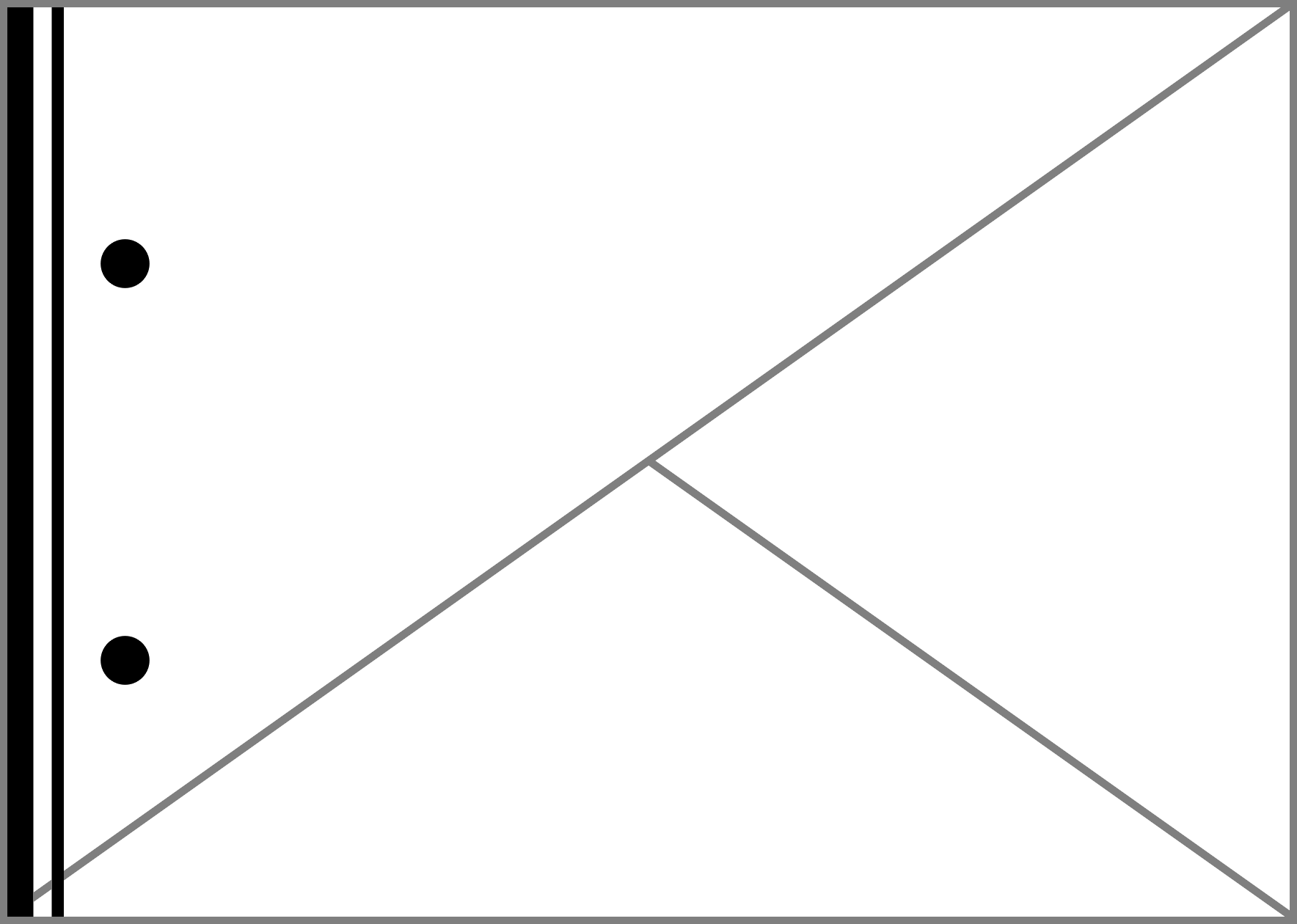X X \e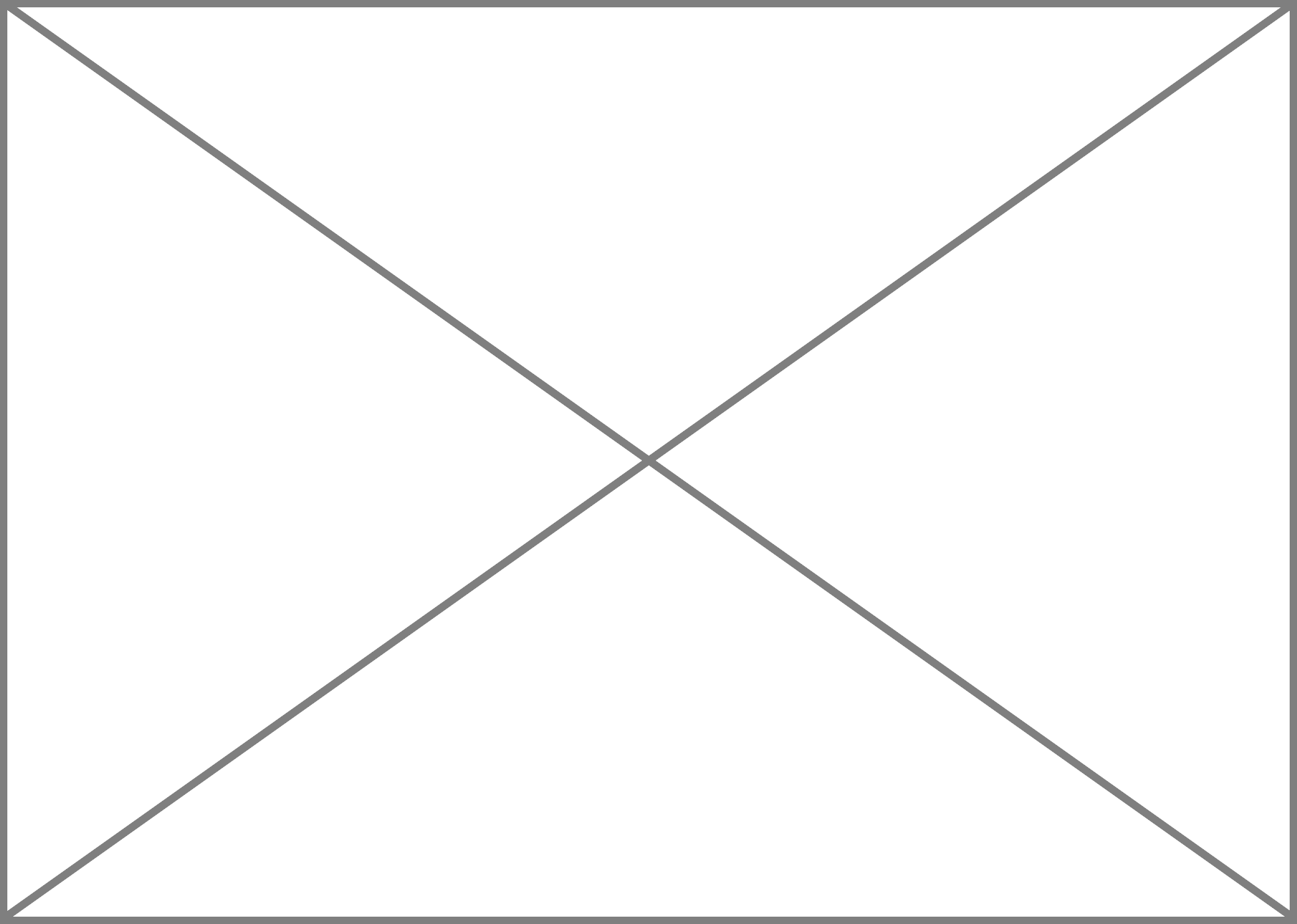\len n \f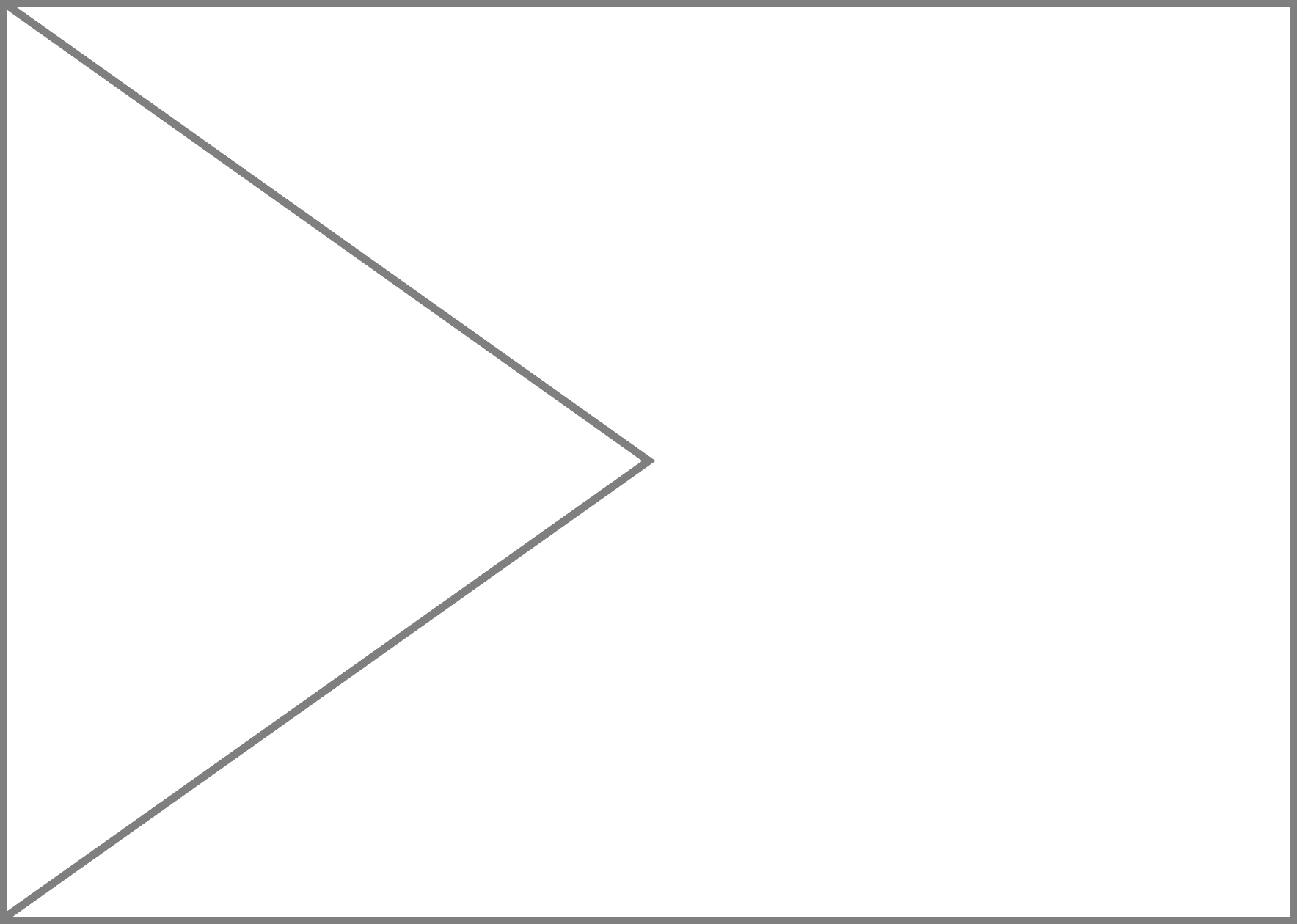\lf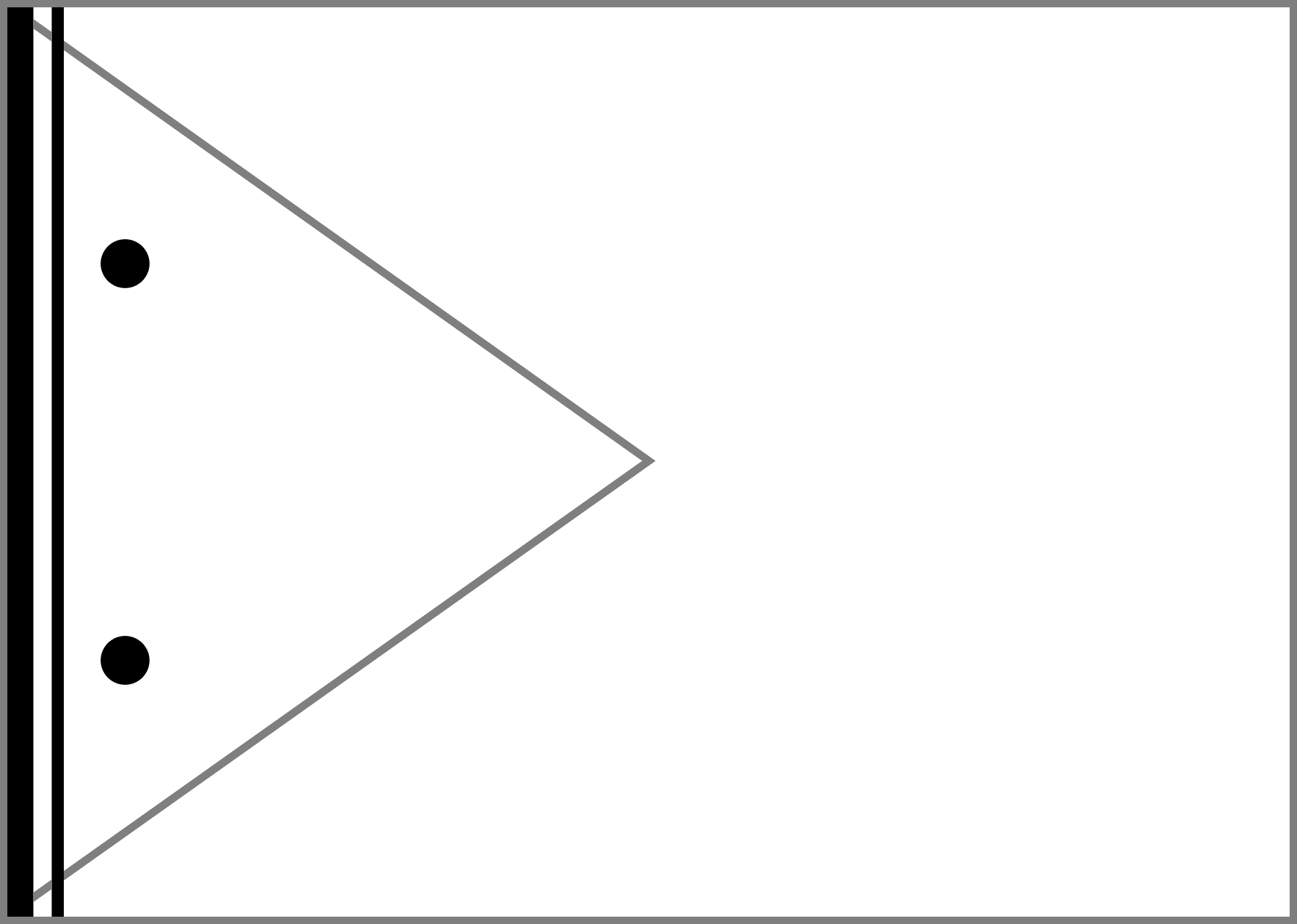v v \g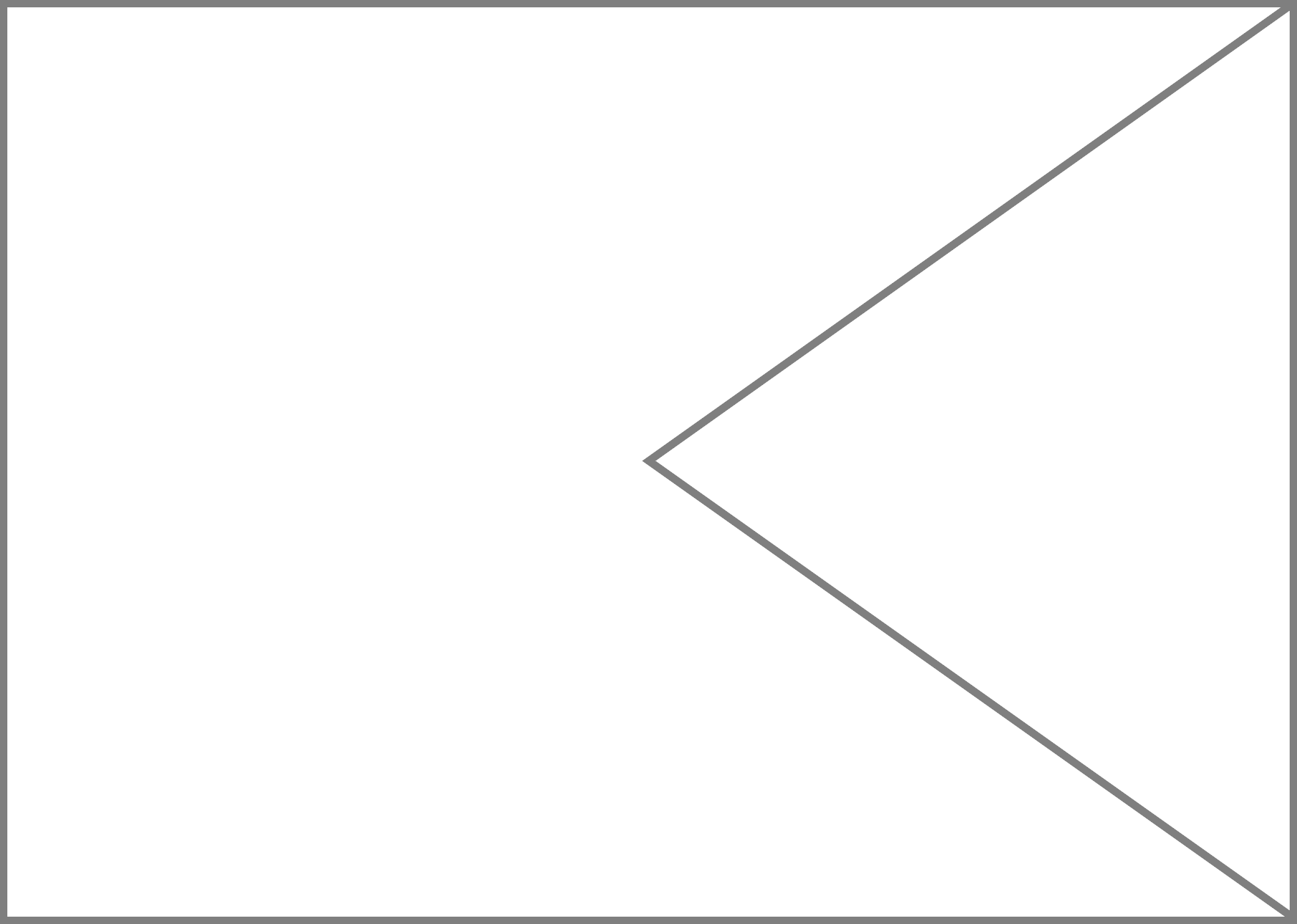\lg% % \fa\ra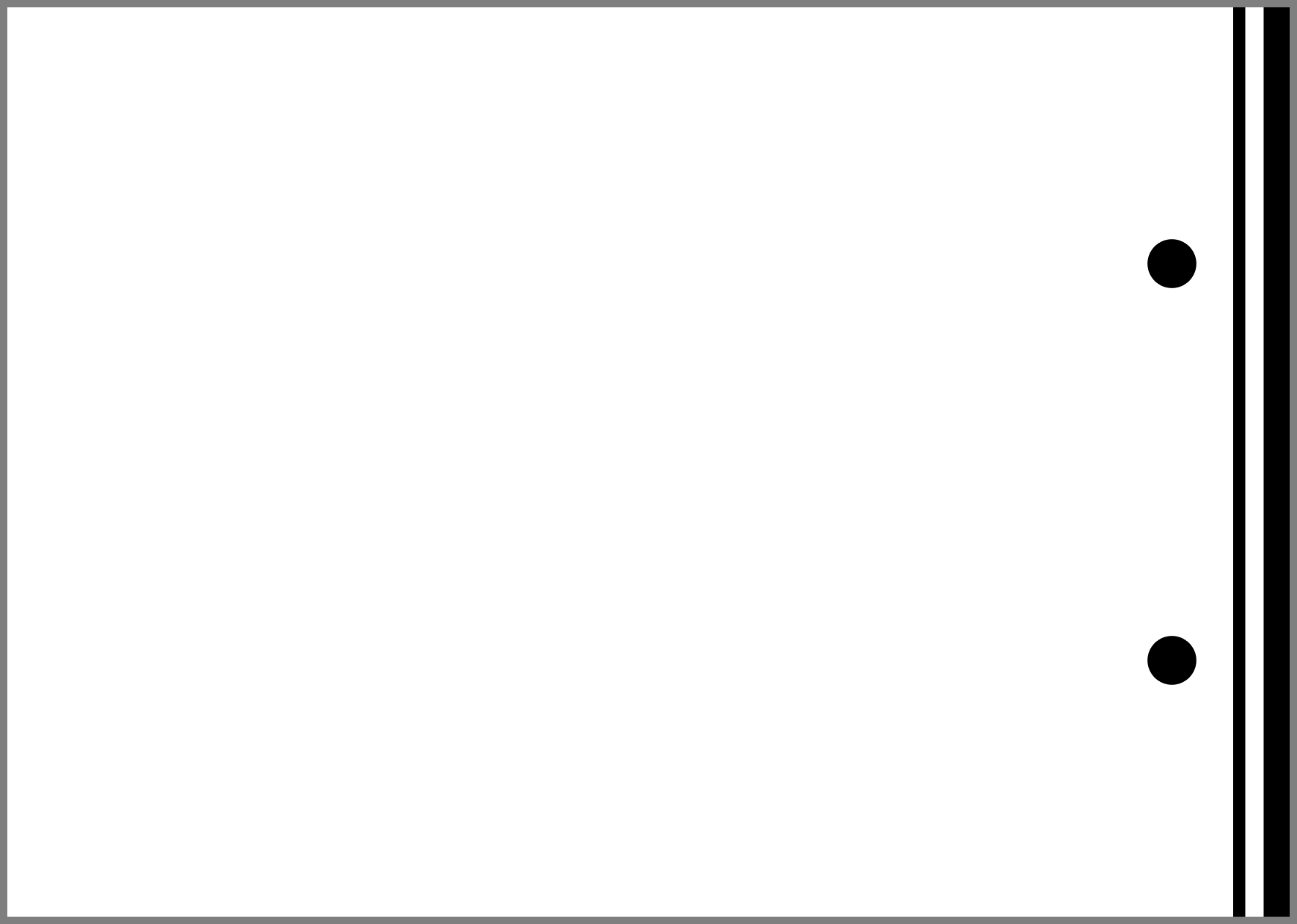Y Y \fb\rbt t \fc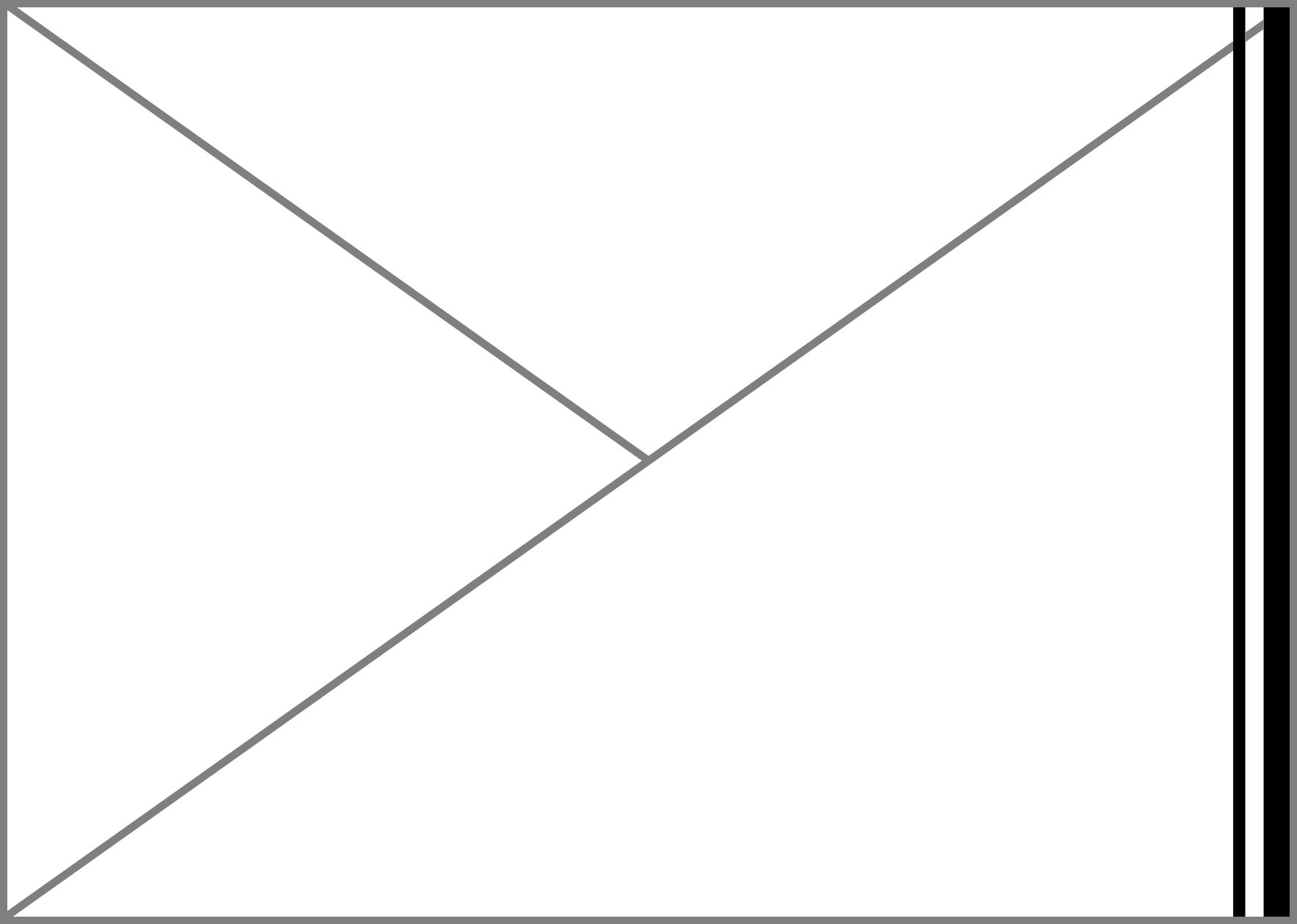\rc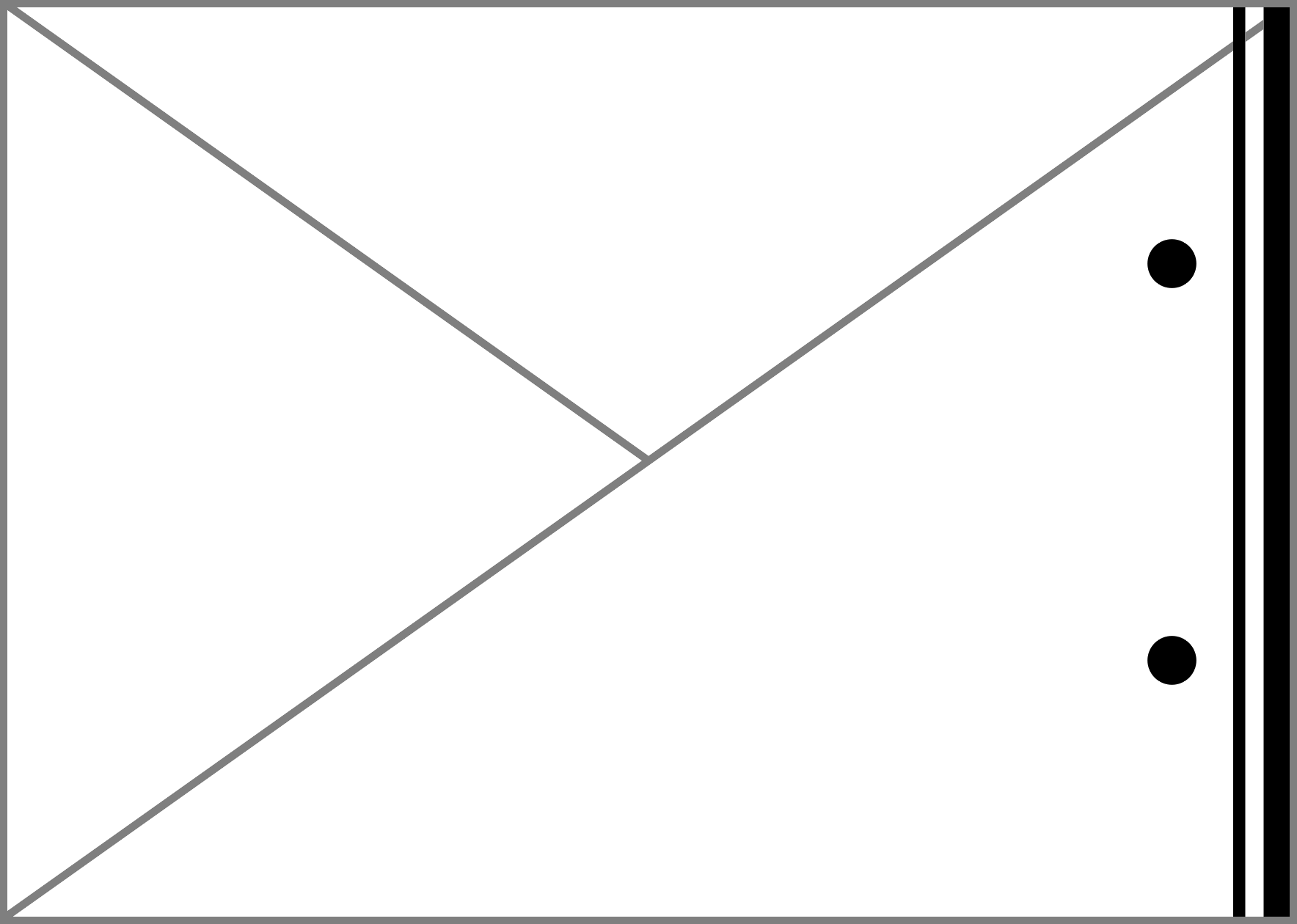s s \fd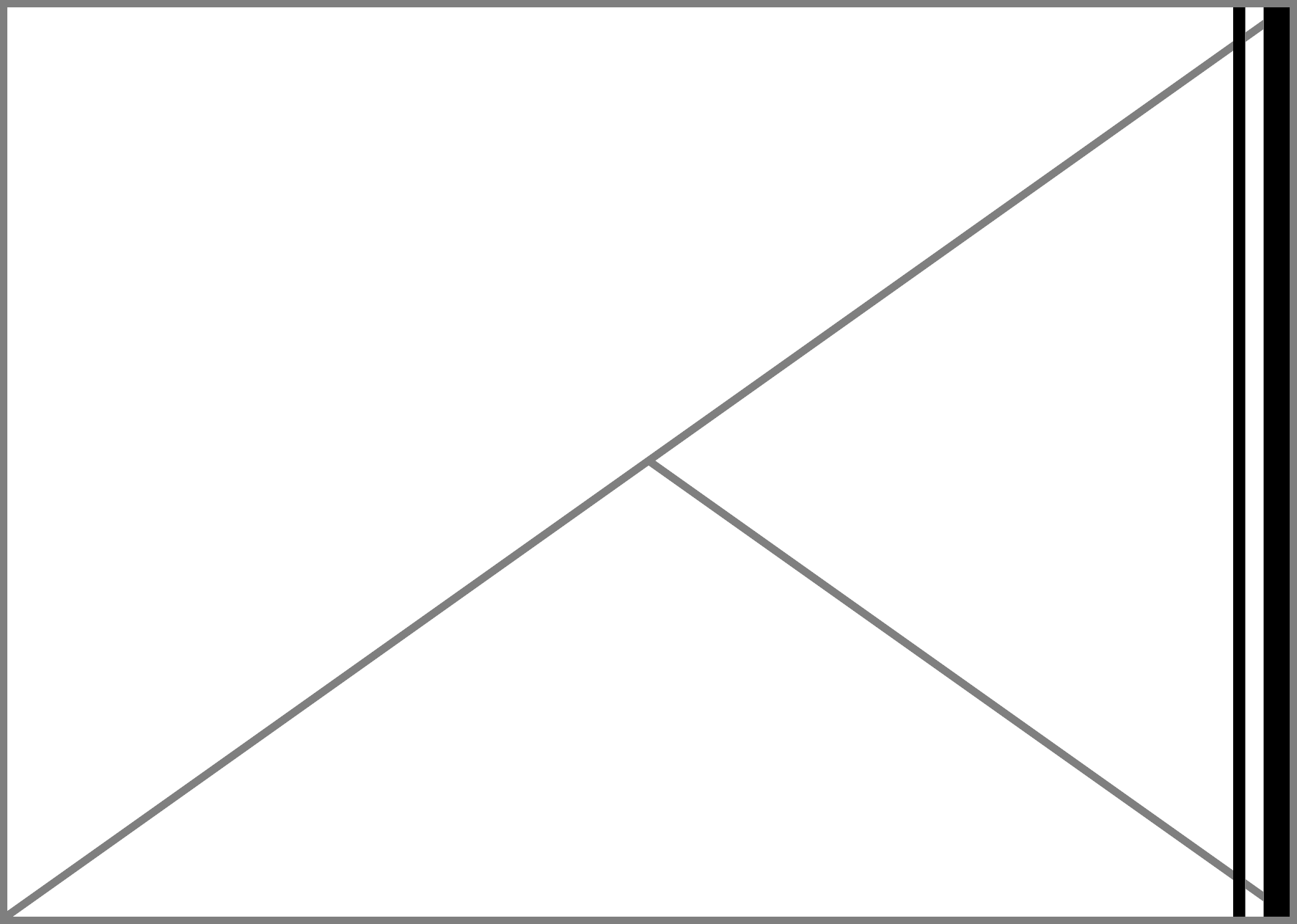\rd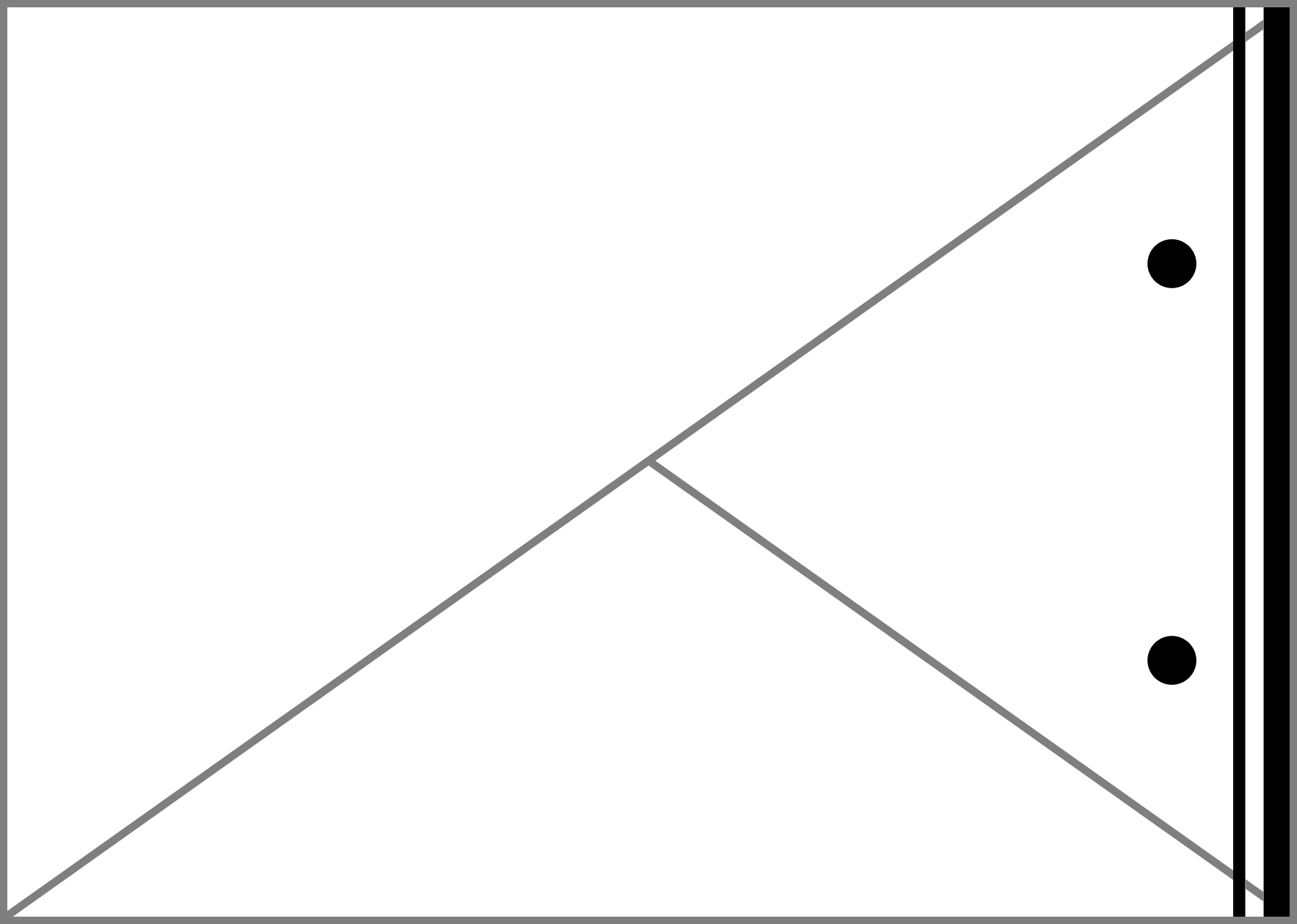h h \fe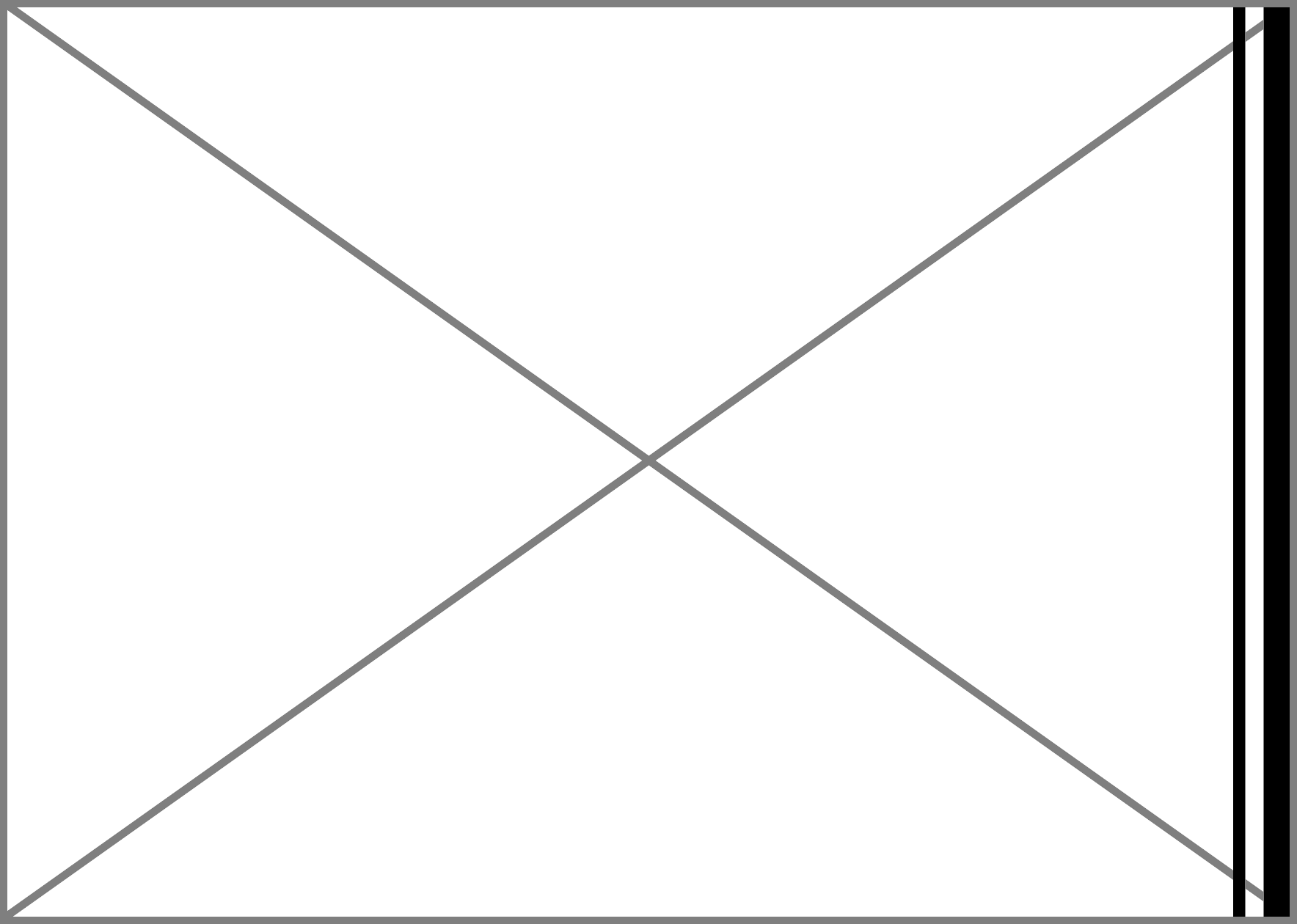\rei i \ff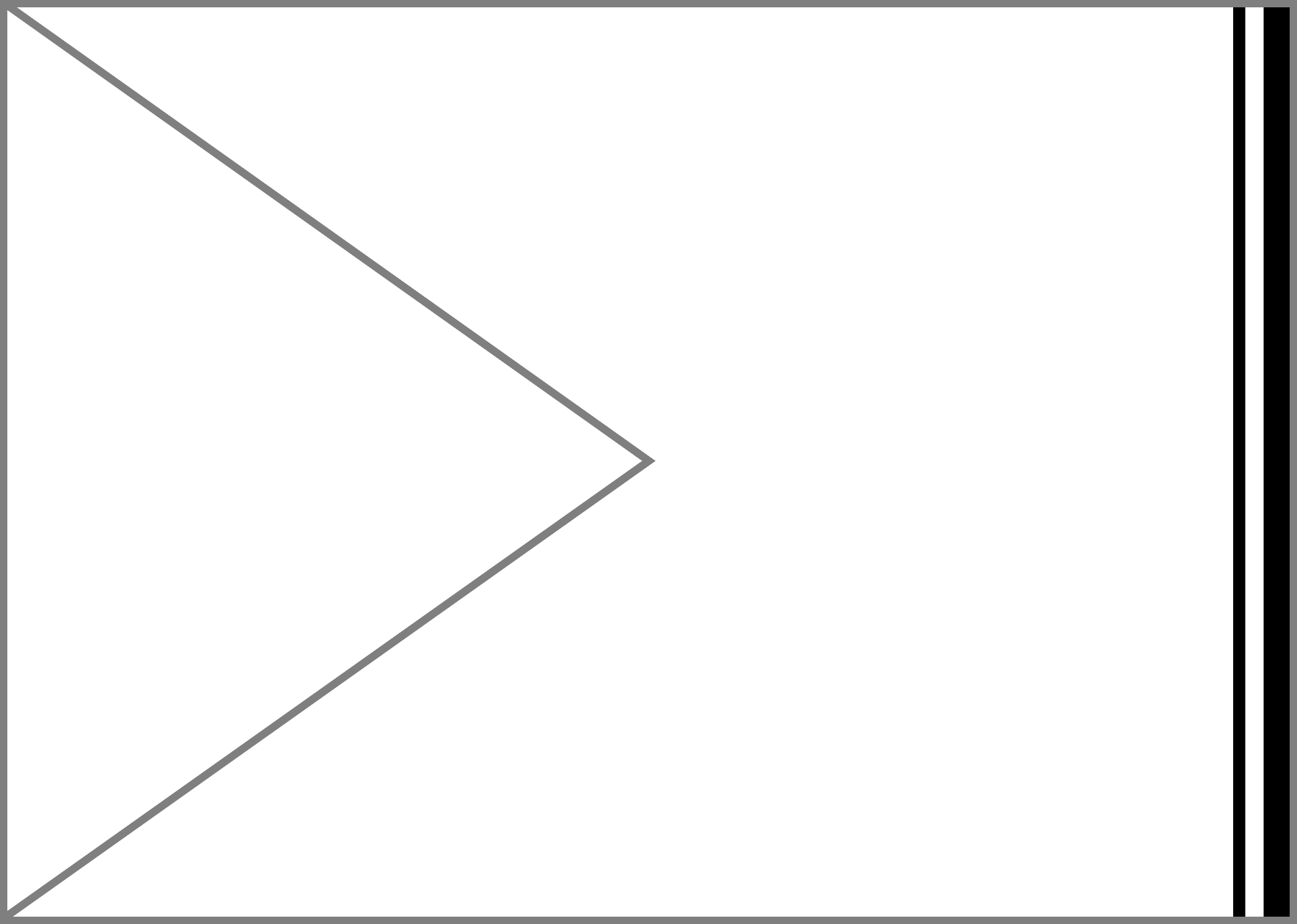\rf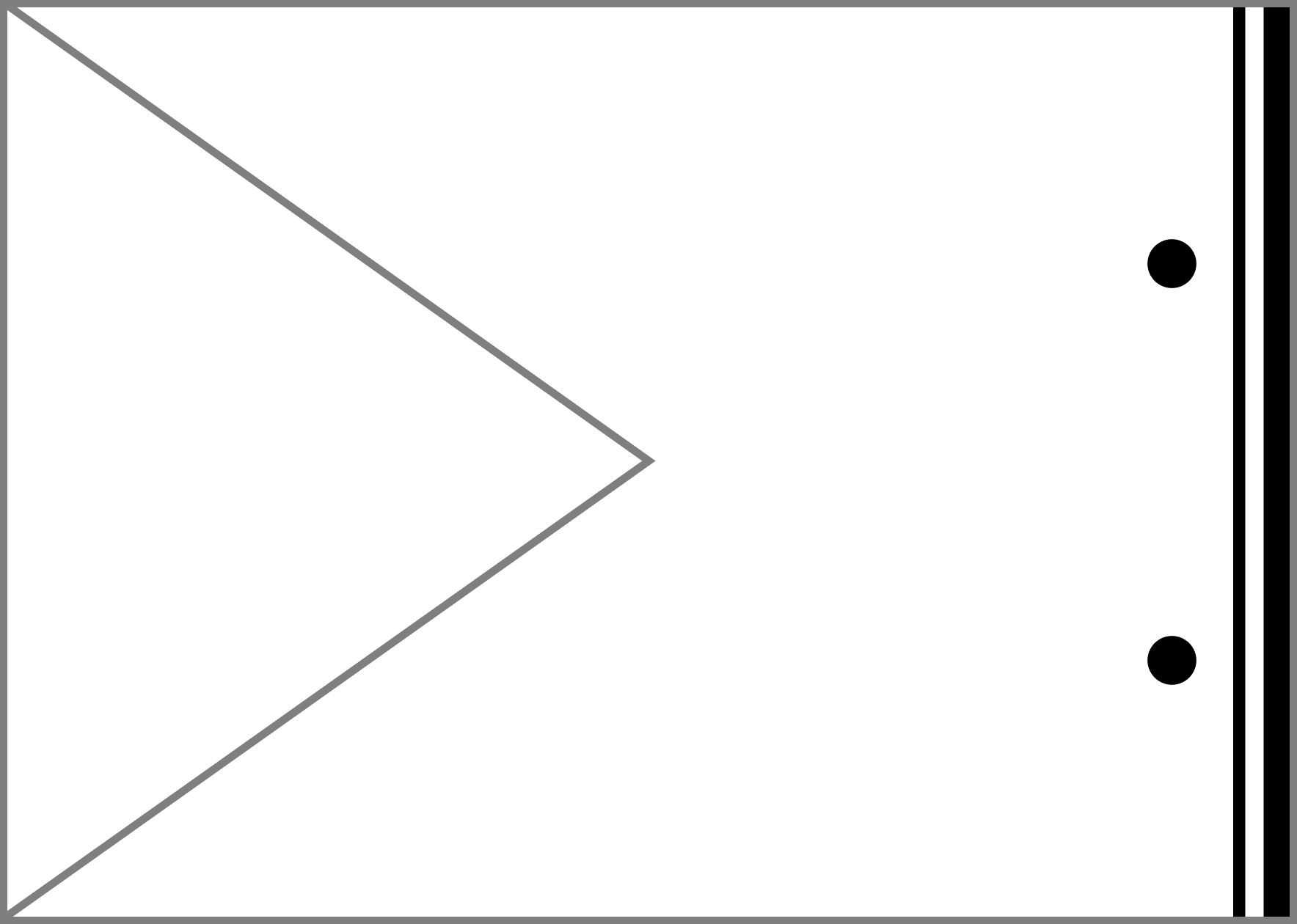j j \fg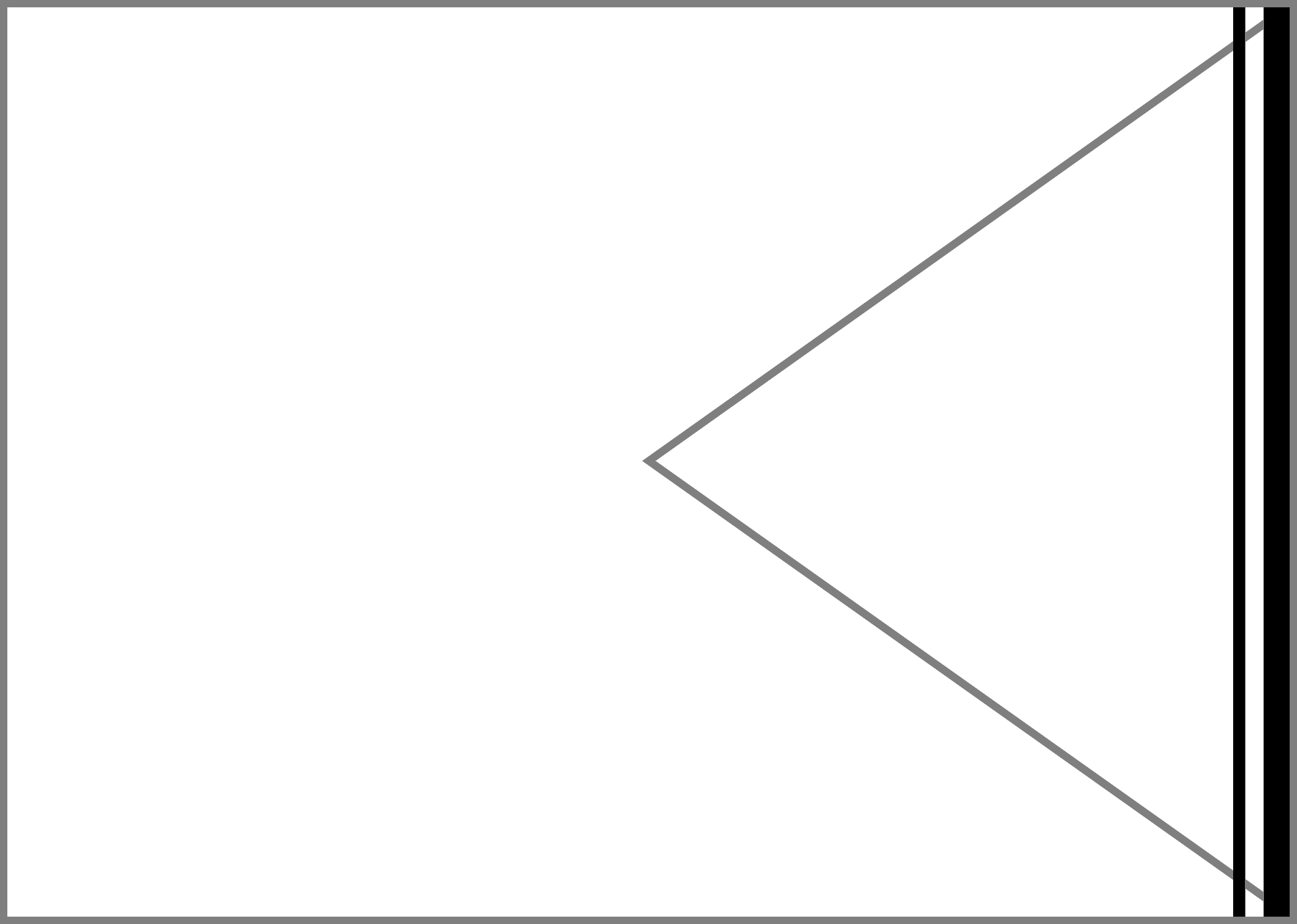\rg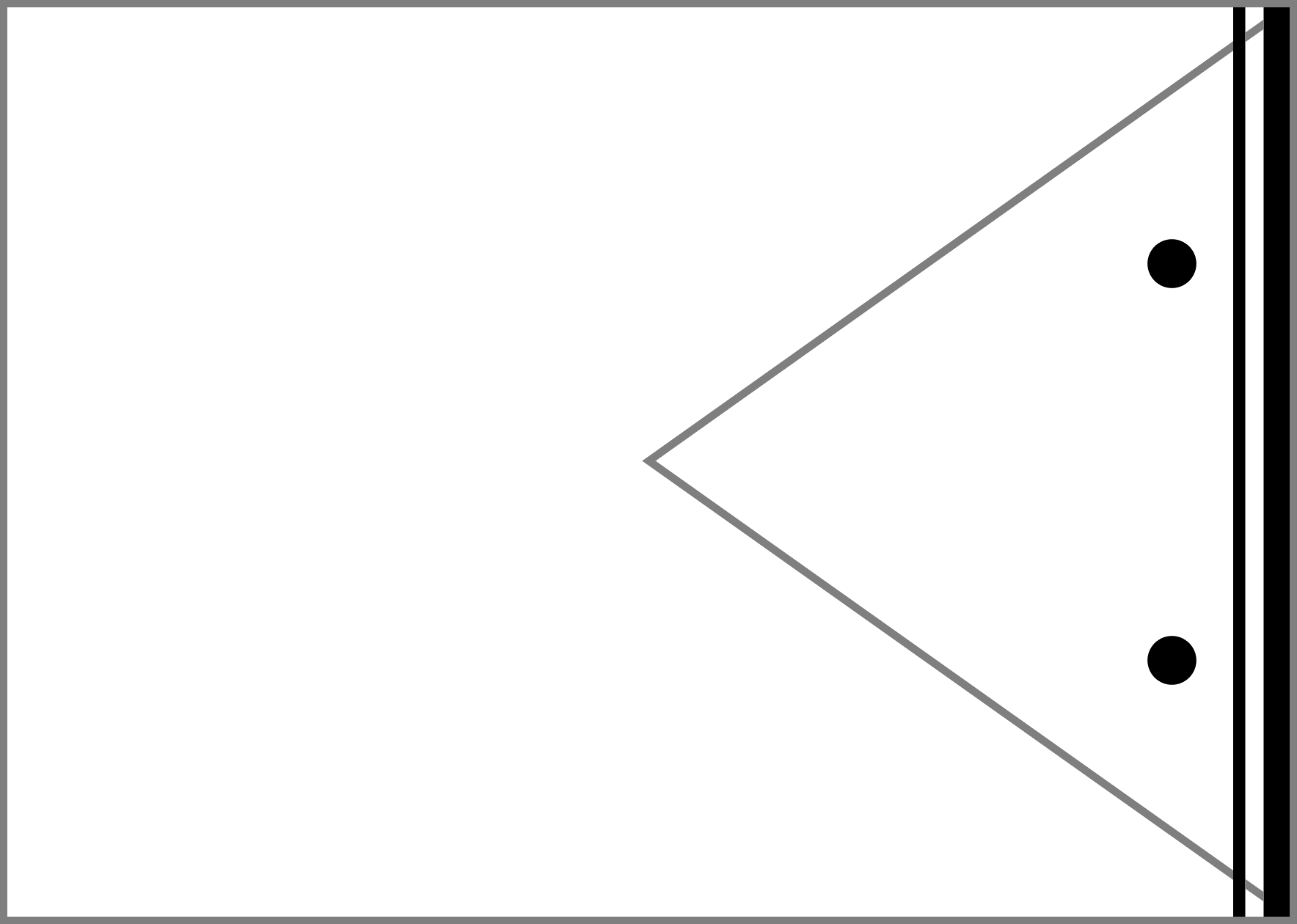k k